### A Critical Statistical Analysis.

By Remi Van Haelst, Belgium
Reprinted by Permission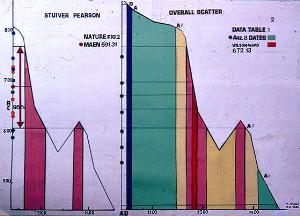The Nature report.
The report "Radiocarbon dating the Turin Shroud", Damon et al, in Nature, (Vol.337, Nr. 6200 pages 611-615. 16 Feb. 1989) concludes:

 Table 1 (Only for the Shroud) d13C Table 2 Ar. 591-+30 690-+35 606-+41 701-+33 -25 646-+31 689-+16* Ox. 795-+65 730-+45 745-+55 -27 750-+30 691-+31' Zu. 733-+61 722-+56 635-+57 639-+45 679-+51 -25.1 676-+24 * = Weighted mean ' = Unweighted mean. X2 = 6.4 %SL. = 5

The reason for a statistical analysis of radiocarbon dates, is the FACT that, theoretically, no experimental result is absolute, and to some extent, subject to chance error. Even an EXACT measurement is still due, to some extent, to chance. Statistical analysis enables the analyst, to determine, with a pre-determined degree of confidence, (here claimed to be at least 95% ) the degree of variations which are due to chance and to known and unknown influences. When applying statistical tests, caution should be observed with data suspected of appreciably differing from a normal distribution.

Examples of distributions:
Normal = Z.  Student = t.  Chi^2 = X^2.  Inverted Beta = F.

The British Museum, selected the Chi^2 to be the criterion for the assessment of the radiocarbon dating results for the Shroud. The MAXIMUM Chi^2 test value for 95% confidence and (3-1) degrees of freedom is 5.99. Theoretically, if the calculated Chi^2 test value could have occurred only by chance, with a probability LESS than that selected, then the set of data would be considered as being DIFFERENT. In practice : Any Chi^2 test value LARGER than 5.99, excludes the claimed 95 % confidence.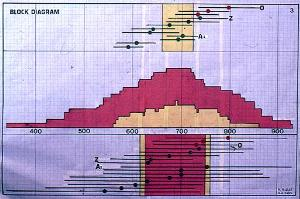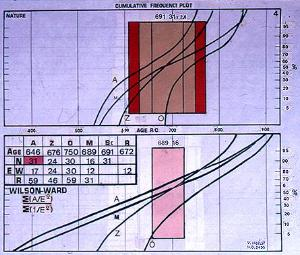First I reworked the data given in Tables 1 & 2, using the classical statistical analyzing method, based on the "Central Limit Theorem." I used the method given in "Perry's Chemical Engineer's Handbook", my technical bible for many years. Note that I only verify the assessment of the radiocarbon dating results, NOT the conversion into calendar ages. All calculations are made on a Sharp PC 4700, using an Ability (Lotus) Spreadsheet. None of the results presented here are biased. Due to rounding up numbers, small differences may occur. For non-mathematical minds, all calculations are worked out completely. The use of complicated formulas is limited to the minimum.

Classical analysis radiocarbon dates for the Shroud of Turin.

 Table 1 Means Table 2 Ar. (591+690+606+701)/4 = 647 F-test = 4.7 > 4.26 = Reject. Ox. (795+730+745)/3 = 757 (647+757+682)/3 = 695 (Un.Mean) Zu. (733+722+639+635+679)/5= 682 (2588+2270+3408)/12 = 689 (W.Mean)

Table 2 Errors based on the scatter of results.

 Ar. {[(591-647)^2+...........+(701-647)^2]/(3x4)}^0.5 = 28 Ox. {[(795-757)^2+(730-757)^2+(745-757)^2]/(2x3)}^0.5 = 20 Zu. {[(733-682)^2+...........+(679-682)^2]/(4x5)}^0.5 = 20 UM. {[(647-695)^2+(757-695)^2+(682-695)^2]/(2x3)}^0.5 = 32 WM. {[(647-689)^2+(757-689)^2+(682-689)^2]/(2x3)}^0.5 = 33

Comparison Table 2 versus Computer.

 Arizona Oxford Zurich U.Mean W.Mean Nature 646-+31 750-+30 676-+24 691-+31 689-+16 Computer 647-+28 757-+20 682-+20 695-+32 689-+33

Because the errors based on the scatter are NOT quoted errors, I did not use the X^2 test, but the more powerful F-test, based on the combination of the 3 means and 12 independent measurements. For 95% confidence, and (3-1) - (12-3) degrees of freedom the maximum F-test, value is 4.26. By chance alone, F should be -+ 1.

F-test :

 Ari Oxf Zur 701 795 733 690 745 722 606 730 679 591 639 635 Sums 2588 2270 3408 = 8266 /4 /3 /5 /12 Mean 647 757 682 689

Between laboratories =2588^2/4+2270^2/3+3408^2/5-8266^2/12=21066
Residual = (701-647)^2+.............(635-682)^2=20167
Total = Sum (Between laboratories + Residual) =41233

Table of variances.

 Source of Variation Sum of Squares Degrees of Freedom Mean Square F Ratio < 4.26 Between 21066 3-1 21066/2=10533 10533/2241 = 4.7 Residual 20167 12-3 20167/9= 2241 Conclusion : Total 41233 11 4.7 > 4.26

Conclusion :
An F-test value of 4.7 > 4.26, states there is a SIGNIFICANT DIFFERENCE between the results given by the three laboratories. In regard to the tabulated data, given in Table 1 of Nature, a certain amount of unexplained variation of the individual runs within and between laboratories is indicated.

Student t distribution test.
The t test compares the sub mean -+error to the final mean. AMS date for 1 run are the mean of about 10 measurements and assuming that the quoted error is equal to the standard error, given in Nature. Because we have to deal with a two tail test, we have to take the critical t value 2.26 for 97.5% confidence and (10-1) degrees of freedom.

A : t-Test versus the sub-means t = < 2.26

 Arizona : 646-+28 Oxford : 757-+20 Zurich : 682-+20 (701-646)/33 = 1.67 (795-757)/65 = 0.58 (733-682)/61 = 0.84 (690-646)/35 = 1.26 (757-745)/55 = 0.22 (722-682)/56 = 0.71 (646-606)/41 = 0.98 (757-730)/45 = 0.60 (682-679)/51 = 0.06 (646-591)/30 = 1.83 (682-639)/45 = 0.96 (682-635)/57 = 0.82

B : t-Test versus the final Mean Final Error = 689-+31 t = < 2.26

 Arizona Oxford Zurich (701-689)/33 = 0.36 (795-689)/65 = 1.63 (733-689)/61 = 0.70 (690-689)/35 = 0.03 (745-689)/55 = 1.02 (722-689)/56 = 0.59 (689-606)/41 = 2.02 (730-689)/45 = 0.91 (689-679)/51 = 0.20 (689-591)/30 = 3.27 (689-639)/45 = 1.11 (689-635)/57 = 0.95

This test indicates that the Arizona date 591-+30 is probably an OUTLIER. Later I tested the individual dates also by a NEW developed test, called the IEM-EEM criterion (Scott et al. 1990). Here the QUOTIENT of the difference between measurement and final mean is divided by the square root of the sum of BOTH the squared errors, it should be < 1.

Here all calculations are based on W & W.

 IEM = < 1 EEM = < 1 Arizona 646-+17 Mean = 672-+13 (701-646)/(17^2+33^2)^0.5 = 1.49 (690-646)/(17^2+35^2)^0.5 = 1.13 (672-646)/(17^2+13^2)^0.5 = 1.21 (646-606)/(17^2+41^2)^0.5 = 0.91 (646-591)/(17^2+30^2)^0.5 = 1.59 Oxford 749-+31 (795-749)/(30^2+65^2)^0.5 = 0.64 (749-745)/(30^2+55^2)^0.5 = 0.06 (749-672)/(30^2+13^2)^0.5 = 2.35 (749-730)/(30^2+45^2)^0.5 = 0.41 Zurich 676-+24 (733-676)/(24^2+61^2)^0.5 = 0.87 (722-676)/(24^2+56^2)^0.5 = 0.75 (679-676)/(24^2+51^2)^0.5 = 0.05 (676-672)/(24^2+13^2)^0.5 = 0.15 (676-639)/(24^2+45^2)^0.5 = 0.73 (676-635)/(24^2+57^2)^0.5 = 0.66

Following the criterion, proposed by Dr. Scott et al, the dates for Arizona (3 x IEM + EEM ) and Oxford (EEM), are OUT of range. Upon meeting Dr. Scott at a Shroud Symposium in New York, I asked her in writing, to verify the Nature dates by the IEM-EEM criterion. But Dr. Scott refused a direct answer.......promising the reply by letter. I am still waiting.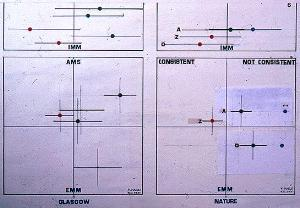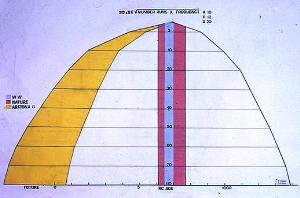I asked the British Museum for some explications. Dr. Morven Leese explained the LOW Arizona errors as follows : Arizona did not send in FOUR, but EIGHT "paired" dates, each pair measured the same day, with the same set of standards and blanks. The EIGHT "dependent" dates were combined in FOUR "independent dates, given in Table 1 (Nature). Each pair of DEPENDENT dates are combined into an INDEPENDENT date.

 A: 606-+41 574-+45 >>>> [606/41^2 + 574/45^2]/[1/41^2 + 1/45^2] = 591 Error = [1/(1/41^2 + 1/45^2)]^0.5 = 30 B: 753-+51 632-+49 >>>> [753/51^2 + 632/49^2]/[1/51^2 + 1/49^2] = 690 Error = [1/(1/49^2 + 1/51^2)]^0.5 = 35 C: 540-+57 676-+59 >>>> [540/57^2 + 676/59^2]/[1/57^2 + 1/59^2] = 606 Error = [1/(1/57^2 + 1/59^2)]^0.5 = 41 D: 701-+47 701-+49 >>>> [701/47^2 + 701/47^2]/[1/47^2 + 1/47^2] = 701 Error = [1/(1/47^2 + 1/47^2)]^0.5 = 33

Yet mathematically correct, this "re-calculation" should have been notified by the authors of the report. At first, the "combination" was silently denied. It took more than TWO years before Arizona confirmed the combination made at the request of the British Museum. The arbitrary "enlarging" of the error from 17 to 31 was never solved. The best results were obtained by averaging the paired errors.
[41 + 45]/2=43 [51 + 49]/2=50 [57 + 59]/2=58 [47 + 47]/2=47
Error on the mean : [1/(1/43^2 + 1/50^2 + 1/58^2 + 1/47^2)]^0.5 = 24.

Based on the scatter, the standard error is 28. At first, I believed that the error was due to the fact, that Arizona included the error in delta 13C at a later stage. The D13C error can be estimated : 31 = [28^2 + (D13C)^2]^0.5
D13C = [961 - 784]^0.5 = 13.

The value appears to be too large. The British Museum gave several versions, but no specific answer was given........Dr. Leese explained also that the British Museum did not use the classical method, but a NEW method, developed by the Australian scientists Drs. Wilson & Ward. (Archeometry 20. 1978). I am still grateful to Drs. Wilson & Ward for their precious help. The method is based on the QUOTED error for each measurement. Quoted errors do incorporate the statistical (counting) error, the error of the scatter of results for standard and blanks, and the (small) uncertainty in the delta 13C determination. Normal statistical and systematic errors are 0.25 %. The total error will be : [0.25^2 + 0.25^2]^0.5 = 0.356 %.

Note : It seems a little strange, that in the W & W method, the LARGER the errors, the BETTER the CHI^2 test will become.

Formula W & W :
Consider a number of measurements -+errors : A-+a........N-+n
Mean X = [A/a^2 + ... + N/n^2]/[1/a^2 + .... + 1/n^2]
Variance: 1/(1/a^2 + ... + 1/n^2)
Error x = [1/(1/a^2 + ...1/n^2)]^0.5
Chi^2 = (A-X)^2/a^2 + ....(N-x)^2/n^2

Calculations :
Arizona :
 701/33^2 + 690/35^2 + 606/41^2 + 591/30^2 2.2241414 -------------------------------------------------- = --------------- = 646 1/33^2 + 1/35^2 + 1/41^2 + 1/30^2 0.0034404 Error = [1/0.0034404]^0.5 = 290.7^2 = 17.05 = 17

Oxford :
 795/65^2 + 745/55^2 + 730/30^2 0.7949403 --------------------------------------- = ------------- = 749 1/65^2 + 1/55^2 + 1/45^2 0.0010609
Error = [1/0.0010609]^0.5 = 942.6^2 = 30.70 = 31

Zurich :
 733/61^2 + 722/56^2 + 679/51^2 + 639/45^2 + 635^2 1.1992731 ------------------------------------------------------------- = ---------- = 676 1/61^2 + 1/56^2 + 1.51^2 + 1/45^2 + 1.57^2 0.0017734
Error = [1/0.0017734]^0.5 = 563.9^2 = 23.75 = 24

Weighted Mean :
 646/17^2 + 749/31^2 + 676/24^2 4.1883016 -------------------------------------- = ----------- = 671.55 = 672 1/17^2 + 1/31^2 + 1/24^2 0.0062368
Error = [1/0.0062368]^0.5 = 160.3^2 = 12.66 = 13.

Note : If we assume, as noted in Nature, that all dates have the same weight, then we can avoid rounding off and calculate the mean -+error as follows :
 2.2241414 + 0.7949403 + 1.1992731 4.2183548 ----------------------------------------- = ------------- = 672 0.0034404 + 0.0010609 + 0.0017734 0.0062747
Error = [1/0.0062747]^0.5 = 159.37^0.5 = 12.62 = 13

X^2 test:
Maximum 5.99 for 95% confidence and (3-1) degrees of freedom.
[(646-672)^2/17^2]+[(749-672)^2/31^2]+(676-672)^2/24^2] = 8.43
The computer, with NO ROUNDING OFF of the values, will give X^2 = 8.76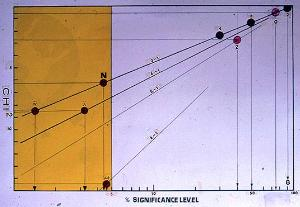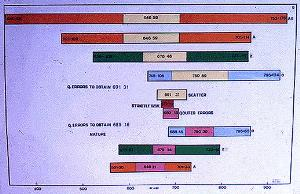Conclusion :
An X^2 test value 8.43 > 5.99 states that there is a SIGNIFICANT DIFFERENCE between the results of the 3 laboratories. From the X^2 test result, one can determinate the % significance level : 2.718^-(8.43/2) = 1.3 %. From this test, one may conclude, that the probability of obtaining, by chance alone, a scatter as high as that observed for the Shroud, is only 13 in 1000. Because we assume all radiocarbon dates to be correct, we must conclude, that the SMALL samples, taken at the same place, do not have the same radioactivity and are not REPRESENTATIVE for the Shroud.

Comparison Table 2 versus the computer.

 Arizona Oxford Zurich Mean Chi^2 S.L.% Nature 646-+31 750-+30 676-+24 689-+16 6.4 5 Computer 646-+17 749-+31 676-+24 672-+13 8.4 1.3

Following the Nature report :
The spread of measurements for sample 1 (Shroud) is somewhat greater than would be expected from the errors quoted. The X^2 test shows that it is UNLIKELY, that the quoted errors FULLY reflect the overall scatter. Not noted is, that a Chi^2 test value > 5.99 DOES EXCLUDE the claimed 95 % confidence. In such cases, one should verify and reject possible "outliers." Possible "outliers" are the Arizona dates 591 and 606, and the Oxford date 795. One may wonder why, the British Museum, did not use the X^2 test for each laboratory.

X^2 test for Arizona (For 4-1 degrees of freedom X^2 = < 7.81)
 (701-646)^2 (690-646)^2 (646-606)^2 (646-591)^2 -------------- + ------------- + ------------ + ---------- = 8.33 > 7.81 33^2 35^2 41^2 30^2

X^2 test for Oxford (For 3-1 degrees of freedom X^2 = <5.99)
 (795-749)2 (749-745)^2 (749-730)^2 ------------- + --------------- + ------------- = 0.68 < 5.99 65^2 55^2 45^2

X^2 test for Zurich (For 5-1 degrees of freedom X^2. = <9.49)
 (733-676)^2 (722-676)^2 (679-676)^2 (676-638)^2 (676-635)^2 -------------- + ------------ + ------------ + ------------ + -------------- = 2.69 61^2 56^2 51^2 45^2 57^2

X^2 for the Mean (For 3-1 degrees of freedom X^2 = <5.99)
 (749-672)^2 (676-672)^2 (672-646)^2 -------------- + ------------- + ------------- = 8.54 > 5.99 31^2 24^2 17^2

The X^2 test indicates INCONSISTENCY for Arizona and the Mean. But the British Museum, on finding "INCONSISTENCY" decided NOT to check for outliers, but to BIAS the results for Arizona by enlarging the error from 17 to 31. This shifts the UNBIASED mean date 672-+13 to 689-+16 and consequently the Chi^2 test value from 8.54 to 6.4 and also the % significance level from 1.3 to 4.4. Finally, it was decided to REPLACE the weighted mean 689-+16 by the UNWEIGHTED mean 691-+31 and to ENLARGE the multiplying factor 1.96 to 2.6, based on an analysis of variance on the 12 individual measurements. The number of degrees of freedom (d), lying between (3-1) inter- and (12-3) intra- laboratory degrees of freedom was ESTIMATED at 5.

Analysis of variance :
 Arizona (701+690+606+591)/4 = 647 Var. (701-647)^2+(690-647)^2+(647-606)^2+(647-591)^2 = 9582 Oxford (795+745+730)/3 = 757 Var. (795-757)^2+(757-745)^2+(757-730)^2 = 2317 Zurich (733+722+679+639+635)/5 = 682 Var. (733-682)62+(722-682)^2+(682-679)^2+(682-639)^2+ (682-635)^2 = 8261

 [9582/4 + 2317/3 + 8261/5]^2 ------------------------------------------------------------------ = ((9582/4)^2)/(4+1) + ((2317/3)^2)/(3+1) + ((8261/5)^2)/(5+1)

 (2395.5 + 722.3 + 1652.2)^2 22752900 -------------------------------------- = ------------ = 13.13 = 13-3 = 10 1147684 + 130429.3 + 454960.8 1733074

The multiplying factor for d=10 and 97.5% confidence = 2.23. (This is for a two tail test and 1 -(2x0,25) = 95 % confidence). An analysis of variance based on 3 laboratories and 16 measurements will only differ for Arizona :

36587/8 = 4573.4 and 4573.4^2/9 = 2323973.1
 [4573.4 + 722.3 + 1652.2]^2 48273314 -------------------------------------- = ------------ = 16.59 = 17-3 = 14 2323973.1 + 130429.3 + 454960.8 2909363

Multiplying the factor for d=14 and 97.5 % confidence = 2.15.

Note : Following the Wilson & Ward method the variance is the squared quoted error.

Strictly following W & W :
 [17^2/4 + 30^2/3 + 24^2/5]^2 ------------------------------------------------------ = 9.22 -3 = 6 [(17^2/4)^2]/5 + [(30^2/3)^2]/4 + [(24^2/5)^2]/6

With Arizona error 31 :
 [31^2/4 + 30^2/3 + 24^2/5]^2 ----------------------------------------------------- = 11.85 - 3 = 9 [(31^2/4)^2]/5 + [(30^2/3)^2]/4 + [(24^2/5)^2]/6

These small differences may seem of little importance, but it is one more indication of a "biased" assessment, with the only purpose of a systematic enlargement of the error range. The same goes for the estimation of the error for the Shroud from the scatter. Here one "forgets" that quoted errors in AMS are deviated from NBS standards and blanks, measured during the SAME run with a series of targets, from the Shroud. Note that for samples 2, 3 and 4, the limits were obtained in the usual way.

This can be illustrated by the Chi^2 and the significance level, given in table 2 (Nature).

 Sample 1 2 3 4 X^2 (2 d.f.) 6.4 0.1 1.2 2.4 Sig.Level % 5 90 50 30

Note : 5% means a 1 chance in 20 that the dates are consistent.

COMMENT :
The advice of Prof. Hoel (University of California) is : "In the case of a X^2 test value close to the limit, it is better not to use such dates in further calculations, but to ask for more and better dates." Despite the use of the NBS standard (Oxalic Acid Standard SRM 4990) the results for the radiocarbon dating of the Shroud show a SYSTEMATIC BIAS and UNEXPLAINED VARIABILITY. An indication of the inhomogeneity of the micro-samples, used in AMS.

Note : Theoretically, all statistical assessments should have been made BEFORE the dC13 correction to -25 o/oo has been applied. In this case the RAW Oxford date would have been -+ 40 years OLDER.

RAW RESULTS.
 Mean : (646/17^2 ) + (790/31^2) + (676/24^2) ----------------------------------------- = 678 -+ 13 1/17^2 +1/31^2 + 1/24^2

 Chi^2 : (646-678)^2 (790-678)^2 (676-678)^2 --------------- + -------------- + -------------- = 17.49 > 5.99 17^2 30^2 24^2

Their can be no doubt, the RAW Oxford results are probably "OUTLIERS." Let us say, that Oxford was saved by the dC13 determination, made IN ANOTHER laboratory.

CONCLUSION :
Facts :

Note :
These calculations have been verified by : Dr. Leese (British Museum). She argues that the differences are caused by the use of different weighting systems! The French professional statisticians Bouclier de Carbon and Forestier, agreed with my results. Dr. Hedges (Oxford) replied : "I do not need statistics....." Dr. Woeffli (Zurich) and Dr. Damon (Arizona) did not reply. Prof. Evin (Lyon. France) : "Statistics will not change the facts...." This paper has been presented at the CIELT International Shroud Symposium. Rome 1993. (Actes CIELT Rome. ISBN 2.868.39.311X Ed. Fr.X. Guibert Paris. pages 207-218).

A REQUEST.
Please use your computer to verify the calculations made in this paper. The W & W method is easily to program on a Lotus Spreadsheet. Let your computer judge the Nature dates and this paper.

Example (Mean -+ error for Arizona):

 A B C D E Age Error (b-)^2 (a-)/(c-) 1/(c-) 1 701 33 1089 701/1089=0.6437098 1/1089=0.0009182 2 690 35 1225 690/1225=0.5632653 1/1225=0.0008153 3 606 41 1681 606/1681=0.3604997 1/1681=0.0005948 4 591 30 900 591/ 900=0.6566666 1/ 900=0.0011111 5 (d5)/(e5) [1/(e5)]^0.5 @sum = 2.2241414 @sum = 0.0034394

a5 = Age = 2.2241414/0.0034294 = 646,6 = 647
b5 = Error = [1/0.0034394]^0.5 = 290.7^0.5 = 17.05 = 17

Repeat this for Oxford, Zurich and calculate the Weighted Mean-+Error from the sub-means-+errors.

 The Chi^2 (X^2) test : Example : (Ar.Mean-W.Mean)^2/Ar.Error^2 (646-672)^2/17^2 = 2.5130111 (Ox.Mean-W.Mean)^2/Ox.Error^2 (749-672)^2/31^2 = 6.1696149 (Zu.Mean-W.Mean)^2/Zu.Error^2 (676-672)^2/24^2 = 0.0277777 Chi^2 = 8.7104037

APPENDIX.

HOW TO BIAS THE COMPUTER RESULTS, TOWARDS 691-+31 ?
The computer results differ TWO times SIGNIFICANTLY from the Nature dates (Table 2).

Mean* = Result with Arizona 646-+17.
Mean\$ = Result with Arizona 646-+31.

 Arizona Mean* Mean\$ Computer 646-+17 672-+13 689-+16 Nature 646-+31 689-+16 691-+31 Factor 31/17 31/16

ESTIMATION OF INDIVIDUAL ERRORS (Table 1) IN FUNCTION OF 691-+31.
First the individual Arizona errors should be multiplied by a factor of 31/17.
Secondly, all individual errors should be multiplied by a factor of 31/16.

Table 1 Individual errors.
 Arizona x 31/17 x 31/16 Oxford x 31/16 Zurich x 31/16 701-+33 60 117 795-+65 126 733-+61 118 690-+35 64 124 745-+55 107 722-+56 108 606-+41 75 145 730-+45 87 679-+51 99 591-+30 55 106 639-+45 87 635-+57 110

Table 2 Errors on the mean.
Arizona error :
[1/(1/117^2 + 1/124^2 + 1/145^2 + 1/106^2)]^0.5 = 60

Oxford error :
[1/(1/126^2 + 1/107^2 + 1/87^2)]^0.5 = 60

Zurich error :
[1/(1/118^2 + 1/108^2 + 1/99^2 + 1/87^2 + 1/110)]^0.5 = 46

Mean age :
 646/60^2 + 750/60^2 + 676/46^2 0.7072483 ------------------------------------- = -------------- = 688 1/60^2 + 1/60^2 1/46^2 0.0012080

Error :      [1/(1/60^2 + 1/60^2 + 1/46^2)]^0.5 = 31

X^2 test :
(689-646)^2/60^2 + (750-689)^2/60^2 + (689-676)^2/46^2 = 1.64
Significance level = 2.718^-(1.64/2) = 44 %

Comparison with X^2 and % Sig.Level.
C = Computer strictly W & W for sample 1.
CC = Computer with errors in function of final error 31.

 Sample C 1 4 CC 3 2 X^2 1.6 6.4 2.4 1.64 1.3 0.1 S.L.% 1.3 5 30 44 50 90

Conclusion : The computer estimated individual errors for the Shroud, in function of error 31 on the unweighted mean 691 are NOT abnormally high and NOT out of range for the claimed AMS accuracy.

A NEW STATISTICAL ASSESSMENT FOR AMS. RADIOCARBON DATES.

Because AMS radiocarbon dating results are NOT absolute values, but the result of a number of X runs, with a frequency Y, all results are given with a certain error. The more measurements closer to the UNKNOWN true value and TRUE error, the closer the averaged and error mean will become. Theoretically, an INFINITE number of measurements will yield a result INFINITELY close to the TRUE value-+ error. Practically, one has to take into account, the precision of the measurement and the systematic errors of the method.

Example : All Oxford sub-errors, lower than 40 have been rounded up to 40.

Following the Nature report, the radiocarbon age of the Shroud, with a 95 % confidence, is 691-+31 years BP. (Before Present = 1950). By backward calculation from this result, the INDIVIDUAL errors can be estimated.

Table 1 in Nature would look like this :

 Arizona x 1.82 591-+ 55 701-+ 60 690-+ 64 606-+ 75 All errors x 1.94 591-+107 701-+116 690-+124 606-+145 (Ar) 795-+126 745-+107 730-+ 87 (Ox) 733-+118 722-+108 679-+ 99 639-+ 87 635-+110 (Zu)

Because THEORETICALLY, any measurement between the limits 606 - 145 = 416 and 795 + 126 = 921 can be the TRUE date, a good estimation of the 95% confidence range can obtained from a DOUBLE statistical analysis based on MINIMUM and MAXIMUM values.

 Minimum Maximum Ari. Oxf. Zur. Ari. Oxf. Zur. 484 669 615 698 921 851 585 638 614 817 852 830 566 643 580 814 817 778 461 552 751 726 525 745 Tot. 2096 1950 2887 3072 2590 3930 Mean 524 650 577 768 863 786 Er. 33 10 18 28 31 29 W.Mean. 578 799 Error 37 30 95 % 578-(2x37)=504 799+(2x30)=859

Any date between those limits 504-859 can be the TRUE date. Here, about 30 % of this age range will be YOUNGER than the HISTORICAL age of 1355 AD.

The Bienayme-Tchebychev formula.
In the case of non normal distributed dates, the error multiplying factors can be estimated, by the Bienayme-Tchebychec inequality.
Probability : {[X-M] < MF X SIGMA} > 1-1/MF^2.

Thus MF for:
68 % = 1-1/1.77^2 = 1-1/3.31 = 1-0.32 = 0.68
75 % = 1-1/2.00^2 = 1-1/4.00 = 1-0.25 = 0.75
90 % = 1-1/3.16^2 = 1-1/10.0 = 1-0.10 = 0.90
95 % = 1-1/4.47^2 = 1-1/20.0 = 1-0.05 = 0.95

The error range for 691-+31 at 95% confidence will be :
691-(4.47x31) = 552 < 691 < 830 = 691+(4.47X31)

Here about 21 % of this age range will be YOUNGER than the HISTORICAL age of 1355 AD. It is clear, that such large errors are unacceptable for the precision claimed for AMS age rc. determinations. (Source Prof. Jouvenoux. University of Marseille-Aix. France).

Top of Page Main Menu Scientific Papers